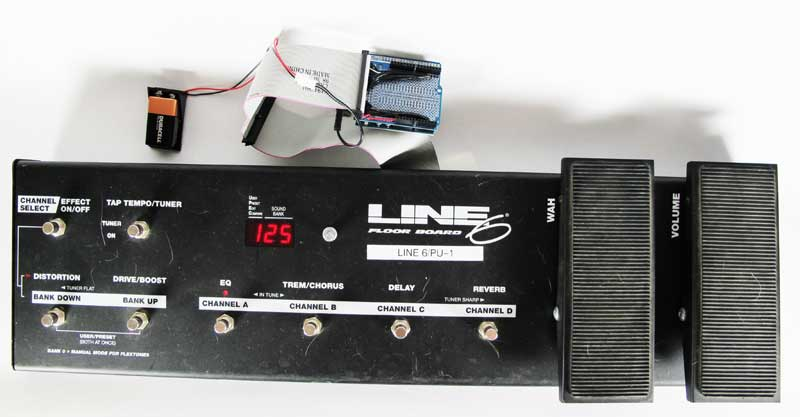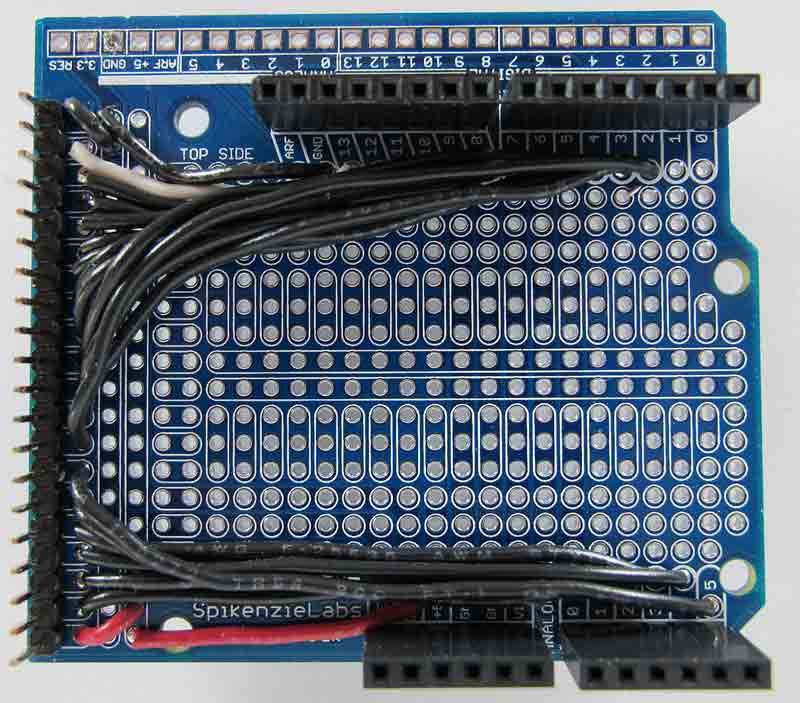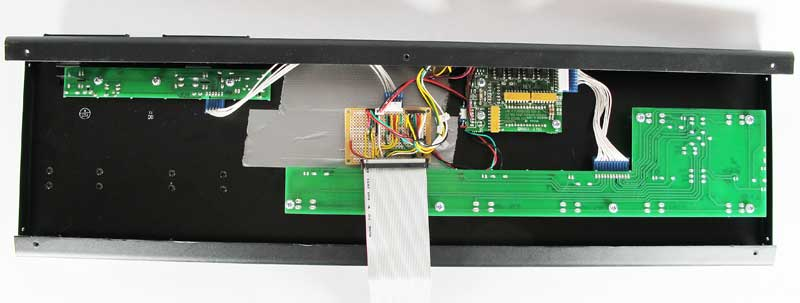# PU-1: Complete V 1.0

The PU-1 is complete, at least in its prototyping phase. The connection board is done and the external Arduino/Shield is attached.In this picture, the PU-1 is powered by a 9 volt battery. At the top is the Arduino with the connection shield and the connection ribbon. I removed the USB connector from this picture. While doing some tests, I also attached the midi-to-USB converter to the Arduino pins through the shield.

The connection board

The connection board is used to assemble the various components that the PU-1 needs to communicate with the rest of the system.At the top of the board, a 20 pin insert is used to connect to a ribbon cable, from an old computer. Luckily, the ribbon is terminated with a connector using standard .1″ spacing. There are 6 pins unused on the 20-pin connector: 3 analog and 3 digital pins. Plenty of room for expansion. At the bottom of this board, from left to right, we have:

With as few electronic parts as possible, this board is pretty much indestructible.

The Arduino and Arduino ShieldI use a Spikenzielab Shield Dock. The 20-pin connector on the left connects to all digital pins on the Arduino, except pins 0 & 1, that are used for serial communication (midi). I could have assembled all the electronics used on the little connection board directly on this shield. I chose to have two boards because this shield will probably be used differently as this is only a prototyping pedal board. The part that stays inside the pedal board will not change that much, except when adding new switches and sensors.

The PU-1

Since I’m using a flat ribbon cable, I can re-install the PU-1 bottom cover over it. I use a layer of insulating tape to protect the ribbon. The grey masking tape under the connection board is replaced with an insulation layer (cardboard!) taped to it. I will eventually screw the board to the top plate.The Arduino Code

Of course, all this works because the Arduino controls the pedal board. This is the code that I’m using during the test stage.

```/*
* First Section: configure the periodical execution of a user
* defined function (Interrupt service routine) using Timer2. This
* example will run the function every 1ms.
*/

#include <Bounce.h>

/* Timer2 reload value, globally available */
unsigned int tcnt2;

int pin1 = 12;
int pin2 = 11;
int counter = 0;
int oldCounter = 0;

// Instantiate a Bounce object with a 5 millisecond debounce time
// Only pin1 needs to be debounced. It is assumed that pin2
// will be stable when reading pin1
Bounce bouncer1 = Bounce( pin1,5 );

/* Second Section:
Use 3 74HC595 shift registers to display 3 numbers on
common cathode 7-segments LEDs, and one more 595 to turn a series of
10 LEDs on and off.
This code should work for any digit assembly, but was specifically
used to recuperate the digits of an old Line 6 floor board.
U1 pin 12 is Latch
U1 pin 11 is Clock
U1 pin 14 is Serial data input.
*/
int LatchPin = 6;
int ClockPin = 5;
int DataPin = 7;
char m;
byte data;
byte dataArray; //character segment matrice
int k = 100; //divider for number display
int showLed = 0x00; //led array
int dp1 = 0x00; //connected to LED 1
int dp2 = 0x00; //connected to LED 2
int dp3 = 0x00; //connected to decimal point on digit 1

//definitions for pedals and their LEDs
int express=1;
int oldExpressValue = 0;
int wah=2;
int oldWahValue = 0;
int swresist=0;
int resistValue;
int showTemp = 0;

//third section: MIDI
// general midi notes
char note1 = 60; //Middle C
char note2 = 62; //D
char note3 = 64; //E
char note4 = 65; //F
char note5 = 67; //G
char note6 = 69; //A
char lastCmd = {0x00,0x00,0x00};

//Fourth Section: General setup
int pin13 = 13;

void setup() {
//setup for the display
pinMode(LatchPin, OUTPUT);
pinMode(ClockPin, OUTPUT);
pinMode(DataPin, OUTPUT);
//setup for the rotary encoder
pinMode(pin1, INPUT);
pinMode(pin2, INPUT);

Serial.begin(31250);

//This section for the Timer Interrupt

/* First disable the timer overflow interrupt while we're configuring */
TIMSK2 &= ~(1<<TOIE2);

/* Configure timer2 in normal mode (pure counting, no PWM etc.) */
TCCR2A &= ~((1<<WGM21) | (1<<WGM20));
TCCR2B &= ~(1<<WGM22);

/* Select clock source: internal I/O clock */
ASSR &= ~(1<<AS2);

/* Disable Compare Match A interrupt enable (only want overflow) */
TIMSK2 &= ~(1<<OCIE2A);

/* Now configure the prescaler to CPU clock divided by 128 */
TCCR2B |= (1<<CS22)  | (1<<CS20); // Set bits
TCCR2B &= ~(1<<CS21);             // Clear bit

/* We need to calculate a proper value to load the timer counter.
* The following loads the value 131 into the Timer 2 counter register
* The math behind this is:
* (CPU frequency) / (prescaler value) = 125000 Hz = 8us.
* (desired period) / 8us = 125.
* MAX(uint8) + 1 - 125 = 131;
*/
/* Save value globally for later reload in ISR */
tcnt2 = 131;

/* Finally load end enable the timer */
TCNT2 = tcnt2;
TIMSK2 |= (1<<TOIE2);
}

/*
* Install the Interrupt Service Routine (ISR) for Timer2 overflow.
* This is normally done by writing the address of the ISR in the
* interrupt vector table but conveniently done by using ISR()  */
ISR(TIMER2_OVF_vect) {
/* Reload the timer */
TCNT2 = tcnt2;

bouncer1.update();
if(bouncer1.risingEdge()){
oldCounter = counter;
counter--;
}
else{
counter++;
}
}

//End of Timer Interrupt section

//Define character images in an array
dataArray = 0x3f; // 0
dataArray = 0x06; // 1
dataArray = 0x5B; // 2
dataArray = 0x4F; // 3
dataArray = 0x66; // 4
dataArray = 0x6D; // 5
dataArray = 0x7C; // 6
dataArray = 0x07; // 7
dataArray = 0x7F; // 8
dataArray = 0x67; // 9
}

void loop()
{
//Read pedal values and show reading from 0 - 127
//need to be calibrated by hand for now. Routine to come
int expressValue = map(analogRead(express),950,85,0,127);
if (expressValue < (oldExpressValue-1) || expressValue > (oldExpressValue+1)) {
showValue(expressValue);
oldExpressValue = expressValue;
midiCmd(0xB0,0x0B, char(expressValue));
}
int wahValue = map(analogRead(wah),950,85,0,127);
if (wahValue < (oldWahValue-1) || wahValue > (oldWahValue+1)) {
showValue(wahValue);
oldWahValue = wahValue;
midiCmd(0xB0,0x07,char(wahValue));
}

//treat resistor value. No switch pressed = 916/1024
//leds 10,8,7 as MSB then6,5,4 and 3 as LSB binaries
//into variable showLed
if ((resistValue > 750) && (lastCmd == char(0x90)) && (lastCmd != 0x00)){ //no pedal pressed -> all notes off
midiCmd(0x90,lastCmd,0x00);
lastCmd = 0x00;
}

//  Serial.println(resistValue);
if (resistValue < 10){
//Tuner ON
showLed = 0x80;
delay(500);
digitalWrite(pin13,HIGH);
else
digitalWrite(pin13,LOW);
}
if (resistValue >= 35 & resistValue < 50){
//Effect control OR Channel Select
//Using LED 1 & 2
delay(500); //wait for switch release. Might need interrupt
if (dp1 == 0x00){
dp1 = 0x80;
dp2 = 0x00;
dp3 = 0x00;
}else {
dp1 = 0x00;
dp2 = 0x80;
dp3 = 0x00;
}
showLed = 0x00;
}
if (resistValue >= 142 & resistValue < 155){
if(lastCmd != note1)
midiCmd(0x90, note1, 0x45);
showLed = 0x01;
}
if (resistValue >= 205 & resistValue < 215){
if(lastCmd != note2)    midiCmd(0x90, note2, 0x45);
showLed = 0x02;
}
if (resistValue >= 270 & resistValue < 290){
if(lastCmd != note3)    midiCmd(0x90, note3, 0x45);
showLed = 0x04;
}
if (resistValue >= 350 & resistValue < 500){
if(lastCmd != note4)    midiCmd(0x90, note4, 0x45);
showLed = 0x08;
}
if (resistValue >= 575 & resistValue < 585){
//Enter special mode. Light left Decimal point
dp1 = 0x00;
dp2 = 0x00;
dp3 = 0x80;
}
if (resistValue >= 640 & resistValue < 650){
if(lastCmd != note5)    midiCmd(0x90, note5, 0x45);
showLed = 0x10;
}
if (resistValue >= 710 & resistValue < 720){
if(lastCmd != note6)    midiCmd(0x90, note6, 0x45);
showLed = 0x20;
}

//Getting ready to display values and indicators
if (counter < 0 || counter > 999)
counter = 0;
if (oldCounter != counter){
showValue(counter);
midiCmdShort(0xC0,counter);
}
oldCounter = counter;
//Show me the number!
liteUpDisplay();
delay(5);

} /////END of LOOP

//Prepare array to cascade down shift registers
void showValue(int showNumber) {
int k = 100;
for (int i = 2; i >= 0; i--)
{
m[i] = showNumber / k;
showNumber = showNumber - (m[i] * k);
k = k / 10;
}
}
//Display 3 digits and one of 3 indicators
void liteUpDisplay(){

digitalWrite(LatchPin, LOW);
//rightmost digit. Use | (or) with 0x80 to light led 2
shiftOut(DataPin, ClockPin, MSBFIRST, dataArray[m] | dp1);
//middle digit. Use | (or) to light led 1
shiftOut(DataPin, ClockPin, MSBFIRST, dataArray[m] | dp2);
//lefmost digit. Use | (or) to light decimal point
shiftOut(DataPin, ClockPin, MSBFIRST, dataArray[m] | dp3);
//leds 10,8,7 then6,5,4 and 3 as MSB and LSB binaries
shiftOut(DataPin, ClockPin, MSBFIRST, showLed);
digitalWrite(LatchPin, HIGH);
}

// Send a MIDI Command with 3 parts
void midiCmd(char cmd, char data1, char data2) {
lastCmd = cmd;
lastCmd = data1;
lastCmd = data2;
Serial.print(cmd, BYTE);
Serial.print(data1, BYTE);
Serial.print(data2, BYTE);
}
// Send a MIDI Command with 2 parts
void midiCmdShort(char cmd, char data1) {
lastCmd = cmd;
lastCmd = data1;
Serial.print(cmd, BYTE);
Serial.print(data1, BYTE);
}```
This entry was posted in Arduino, Pedal board and tagged , . Bookmark the permalink.